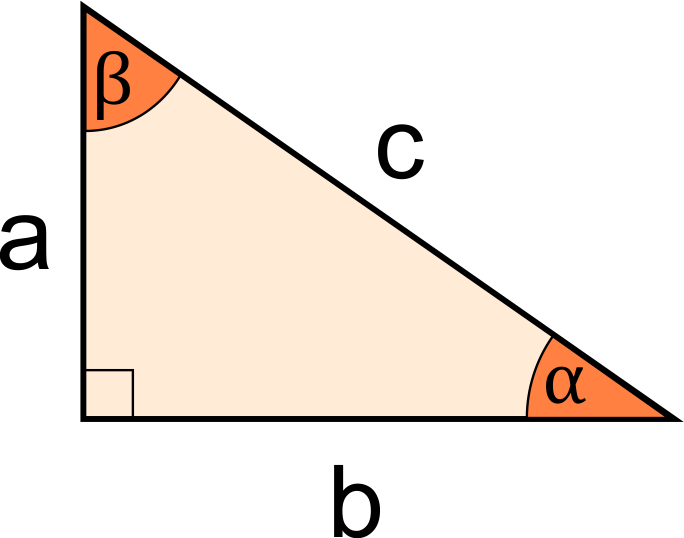# Missing Side of a Right Triangle Calculator

Created by Rahul Dhari
Reviewed by Kenneth Alambra
Last updated: May 28, 2022

The missing side of a right triangle calculator will help you find the third side or any third angle for a right-angled triangle.

A triangle is right-angled if one of the three angles is 90°, i.e., any two sides are perpendicular. In this calculator, we use this property to deliver a calculator that enables you to find the missing side of a right triangle. Read on to understand how to find the missing side of a right triangle.

## Missing side of a right triangle

The formula that relates the sides of the triangle to its angles is the sine law. For a triangle having three sides as $a$, $b$, $c$, and the angles $\alpha$, $\beta$, and $\gamma$, it is given by the equation:

$\frac{a}{\sin{\alpha}} = \frac{b}{\sin{\beta}} = \frac{c}{\sin{\gamma}}$

But we know that one of the angles is 90°. Therefore, the sum of the other two angles is 90°. Mathematically, if $\gamma = 90°$,

$\alpha + \beta = 90° (\text{or } \frac{\pi}{2} \text{ radians})$

Using the above relation, we get:

$\frac{a}{\sin{\alpha}} = \frac{b}{\sin{\beta}} = c\\\\ \frac{a}{\sin{\alpha}} = \frac{b}{\sin({90 - \alpha})} = c$

The above relationship, along with the Pythagoras theorem, forms the core of this tool and is used to calculate the missing sides or angles for any right-angled triangle.

## Using the missing side of a right triangle calculator

Use the calculator to find the missing side of a right triangle having sides 9 and 12.

1. Enter the length of the first side as 9.
2. Insert the length of the second side as 12.
3. The calculator will return the third side length as 15.
4. We can also get the angles for the triangle as 36.87° and 53.13° using the missing side of a right triangle calculator.

Following are the other similar triangle-based tools that might generate your interest:

## FAQ

### How do I find the missing side of a right triangle?

To find the missing side of a triangle:

1. Divide the length of the first side by the second side.
2. Find the inverse tangent of the quotient to get the angle between the two sides.
3. Divide the length of the first side by sine of the angle to obtain the third side of the triangle.

### What is the third side of a triangle having sides, 3 and 4?

The third side of the triangle is 5. First, we find the angle between the two sides, i.e., arctan (3/4) = 36.87°. Then we divide the first side by the sine of angle obtained: 3/sin(36.87°) = 5.

Rahul DhariSides
a
in
b
in
c
in
Angles
Angle α
deg
Angle β
deg
People also viewed…

### Alien civilization

The alien civilization calculator explores the existence of extraterrestrial civilizations by comparing two models: the Drake equation and the Astrobiological Copernican Limits👽

### Inequality to interval notation

Use our inequality to interval notation calculator whenever you need to convert between inequalities and intervals. It is a two-way converter!

### Latus rectum

Find the length of the latus rectum of a parabola, hyperbola, or ellipse with our latus rectum calculator.

### Secretary problem (Valentine's day)

Use the dating theory calculator to enhance your chances of picking the best lifetime partner.